• 本程序是两圆相交求交点，输入信息为圆的圆心坐标和半径值。如有更好的算法，欢迎交流！！！！！！！！！！！！！！！！！！！！！！！！！！！！！！！！！！！！！！！！！！！！！！！！！！！！！！！！！！！...
• 使用java求圆相交的坐标，下载前请参见博客：http://blog.csdn.net/u013780605/article/details/52673223，文章中有具体的过程，该资源为工程文件，可直接编译运行。
• 给定2圆的圆心坐标和半径，计算并输出两圆相交的面积，如果是外切或不相交，则输出0，圆周率取函数值acos(-1)。 输入格式: 输入6个整数x1 y1 r1 x2 y2 r2，分别表示圆的圆心(x1,y1),(x2,y2)和半径r1,r2。 输出格式...
题目
给定2圆的圆心坐标和半径，计算并输出两圆相交的面积，如果是外切或不相交，则输出0，圆周率取函数值acos(-1)。
输入格式:
输入6个整数x1 y1 r1 x2 y2 r2，分别表示两圆的圆心(x1,y1),(x2,y2)和半径r1,r2。
输出格式:
根据圆的位置关系，输出其相交部分的面积（保留2位小数）。
输入样例:
在这里给出2组输入。例如： 0 0 1 2 2 1 2 0 2 5 2 3
输出样例:
在这里给出相应的输出。例如： 0.00 3.22
//此处PI值为acos(-1)
//扇形面积 α * r * r / 2
//求角度cos(A) = (b * b + c * c - a * a) / (2 * b * c)
#include <bits/stdc++.h>

using namespace std;
typedef long long ll;
#define lowbit(x) ((x) & (-x))
#define PI acos(-1)
const int inf = 0x3f3f3f3f;
const int N = 500010;

struct circle {
int x, y, r;
};

double dis(circle a, circle b) {
return sqrt((a.x - b.x) * (a.x - b.x) + (a.y - b.y) * (a.y - b.y));
}

int main() {
#ifndef ONLINE_JUDGE
freopen("in.txt", "r", stdin);
freopen("out.txt", "w", stdout);
#endif
circle a, b;
cin >> a.x >> a.y >> a.r;
cin >> b.x >> b.y >> b.r;
double len = dis(a, b);
if (len + min(a.r, b.r) <= max(a.r, b.r)) {
if (a.r < b.r)
printf("%.2f", PI * a.r * a.r);
else
printf("%.2f", PI * b.r * b.r);
} else if (len >= a.r + b.r) {
cout << "0.00";
} else {
double d1 = 2 * acos((a.r * a.r + len * len - b.r * b.r) / (2 * a.r * len));
double d2 = 2 * acos((b.r * b.r + len * len - a.r * a.r) / (2 * b.r * len));
double area1 = d1 * a.r * a.r / 2 - a.r * a.r * sin(d1) / 2;
double area2 = d2 * b.r * b.r / 2 - b.r * b.r * sin(d2) / 2;
printf("%.2f", area1 + area2);
}

return 0;
}

展开全文• ## 计算两圆相交面积

万次阅读 多人点赞 2018-08-16 18:44:20
转自：[模板] 求两圆相交面积（模板）  两圆相交分如下集中情况：相离、相切、相交、包含。 设圆圆心分别是O1和O2，半径分别是r1和r2，设d为圆心距离。又因为圆有大有小，我们设较小的圆是O1。 相离相切的...
转自：[模板] 求两圆相交面积（模板）
两圆相交分如下集中情况：相离、相切、相交、包含。
设两圆圆心分别是O1和O2，半径分别是r1和r2，设d为两圆心距离。又因为两圆有大有小，我们设较小的圆是O1。
相离相切的面积为零，代码如下：
double d = sqrt((a.x-b.x)*(a.x-b.x) + (a.y-b.y)*(a.y-b.y));
if (d >= r1+r2)
return 0;

包含的面积就是小圆的面积了，代码如下：
if(r2 - r1 >= d)
return pi*r1*r1;

接下来看看相交的情况。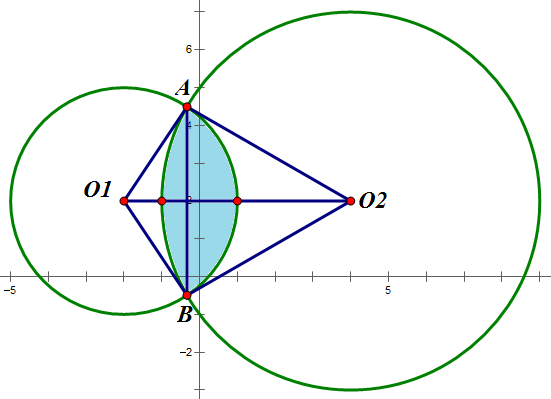相交面积可以这样算：扇形O1AB - △O1AB + 扇形O2AB - △O2AB，这两个三角形组成了一个四边形，可以用两倍的△O1AO2求得，
所以答案就是两个扇形-两倍的△O1AO2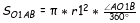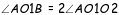因为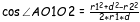所以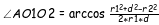那么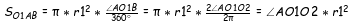同理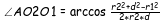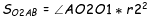接下来是四边形面积：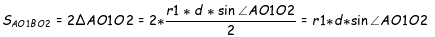代码如下：
double ang1=acos((r1*r1+d*d-r2*r2)/(2*r1*d));
double ang2=acos((r2*r2+d*d-r1*r1)/(2*r2*d));
return ang1*r1*r1 + ang2*r2*r2 - r1*d*sin(ang1);

至此完整代码就可以写出来了：
#include<iostream>
#include<cmath>
using namespace std;

#define pi acos(-1.0)

typedef struct node
{
int x;
int y;
}point;

double AREA(point a, double r1, point b, double r2)
{
double d = sqrt((a.x-b.x)*(a.x-b.x) + (a.y-b.y)*(a.y-b.y));
if (d >= r1+r2)
return 0;
if (r1>r2)
{
double tmp = r1;
r1 = r2;
r2 = tmp;
}
if(r2 - r1 >= d)
return pi*r1*r1;
double ang1=acos((r1*r1+d*d-r2*r2)/(2*r1*d));
double ang2=acos((r2*r2+d*d-r1*r1)/(2*r2*d));
return ang1*r1*r1 + ang2*r2*r2 - r1*d*sin(ang1);
}

int main()
{
point a, b;
a.x=2, a.y=2;
b.x=7, b.y=2;
double result = AREA(a, 3, b, 5);
printf("%lf\n", result);
return 0;
}


展开全文• 无所谓程序设计语言，不过是表达形式不一罢了题目来源理论知识参考知识：代码实现C#鼠标悬停此处预览测试样例JavaKotlinC++CPythonJavaScriptHtmlobjective-cshellperlruby汇编语言 ...四边形面积可由对称的个...


无所谓程序设计语言，不过是表达形式不一罢了
题目来源理论知识参考知识：

代码实现C#鼠标悬停此处预览测试样例
Java鼠标悬停于此预览测试样例
KotlinC++CPythonJavaScriptHtmlobjective-cshellperlruby汇编语言
拓展改成两个圆环以后呢？

题目来源
鼠标悬停于此可预览题目
东华大学 .NET 技术课程中期作业。
帮别人忙，自己昨夜写的一个小程序。


理论知识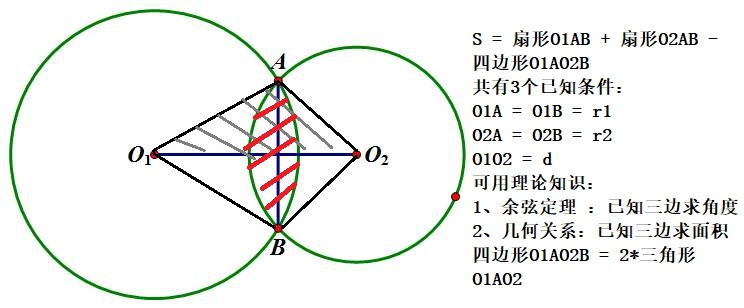如上图所示：
四边形面积可由对称的两个三角形求和而得：
O1AO2B = O1AO2 + O1BO2 = 2 * O1AO2
以三角形 O1AO2 进行分析：
已知三边可以求：任意内角
∠AO1O2 = 余弦定理公式变形可求
该三角形面积 = 多种求法，介绍两种如下：
S = （1.0/2）* 两边乘积*夹角正弦值
或者海伦公式
p = (1.0/2)*(a+b+c)
s = sqrt(p*(p-a)*(p-b)*(p-c))


参考知识：
计算两圆相交面积 全网总结最全：17种求三角形面积的公式，从平面到立体
代码实现

C#
CircleArea.cs文件内容：

using System;

namespace AreaCalculation
{
public class CircleArea
{
private double r1;
private double r2;
private double d;

public CircleArea()
{
this.r1 = .0;
this.r2 = .0;
this.d = .0;
}
public CircleArea(double r1 = .0,  double r2 = .0, double d = .0)
{
this.r1 = r1;
this.r2 = r2;
this.d = d;
}

public void Init(ref double r1, ref double r2, ref double d)
{
this.r1 = r1;
this.r2 = r2;
this.d = d;
}

public double IntersecArea()
{
var area = .0;

if (this.r1 + this.r2 <= d) //两圆外离（切）
{
//return .0;
area = .0;
}
else if (Math.Abs(this.r1 - this.r2) >= d) //两圆内含（切）（此处必须要有等号，要处理同心圆的特例情形）
{
//return (this.r1 < this.r2) ? Math.PI * this.r1 * this.r1 : Math.PI * this.r2 * this.r2;
area = (this.r1 < this.r2) ? Math.PI * this.r1 * this.r1 : Math.PI * this.r2 * this.r2;
}
else //两圆相交
{
double ang1 = Math.Acos((this.r1 * this.r1 + this.d * this.d - this.r2 * this.r2) / (2 * this.r1 * this.d)); //弧度制，用于计算扇形面积（所占圆的比例）
double ang2 = Math.Acos((this.r2 * this.r2 + this.d * this.d - this.r1 * this.r1) / (2 * this.r2 * this.d));
//return ang1 * this.r1 * this.r1 + ang2 * this.r2 * this.r2 - this.r1 * d * Math.Sin(ang1); //两圆相交时，相交面积 = 两扇形面积 - 两圆心与两交点所组成的四边形的面积
area =  ang1 * this.r1 * this.r1 + ang2 * this.r2 * this.r2 - this.r1 * d * Math.Sin(ang1); //两圆相交时，相交面积 = 两扇形面积 - 两圆心与两交点所组成的四边形的面积
}

return area;
}

public void OutResult()
{
Console.WriteLine("两个圆的半径分别为： {0} 和 {1}， 圆心距是：{2}", this.r1, this.r2, this.d);
Console.WriteLine("两个圆所相交的面积为:{0}",Math.Round(IntersecArea(),2));
}
}
}

鼠标悬停此处预览测试样例

Java
// 即将用于和工厂方法实现实例化进行对比
public class CircleArea {

private double _r1;
private double _r2;
private double _d;

public double get_r1() {
return _r1;
}

public double get_r2() {
return _r2;
}

public double get_d() {
return _d;
}

public void set_r1(double _r1) {
this._r1 = _r1;
}

public void set_r2(double _r2) {
this._r2 = _r2;
}

public void set_d(double _d) {
this._d = _d;
}

CircleArea(){
this._r1 = .0;
this._r2 = .0;
this._d = .0;
}
CircleArea(double r1, double r2, double d)
{
this._r1 = r1;
this._r2 = r2;
this._d = d;
}

public double IntersecArea()
{
if (this._r1 + this._r2 <= this._d) //两圆外离（切）
{
return .0;
}
else if (Math.abs(this._r1 - this._r2) >= this._d) //两圆内含（切）（此处必须要有等号，要处理同心圆的特例情形）
{
return (this._r1 < this._r2 ? Math.PI * this._r1 * this._r1 : Math.PI * this._r2 * this._r2);
}
else //两圆相交
{
double ang1 = Math.acos((this._r1 * this._r1 + this._d * this._d - this._r2 * this._r2) / (2 * this._r1 * this._d)); //弧度制，用于计算扇形面积（所占圆的比例）
double ang2 = Math.acos((this._r2 * this._r2 + this._d * this._d - this._r1 * this._r1) / (2 * this._r2 * this._d));
return ang1 * this._r1 * this._r1 + ang2 * this._r2 * this._r2 - this._r1 * _d * Math.sin(ang1); //两圆相交时，相交面积 = 两扇形面积 - 两圆心与两交点所组成的四边形的面积
}
}

public void OutResult()
{
System.out.printf("两个圆的半径分别为： %f 和 %f%n圆心距是：%f\n", this._r1, this._r2, this._d);//%n和\n都可以实现换行功能
System.out.format("两个圆所相交的面积为：%.2f",IntersecArea());
}
}

鼠标悬停于此预览测试样例

Kotlin
待更


C++
待更


C
待更


Python
待更


JavaScript
待更


Html
待更


objective-c
待更


shell
待更


perl
待更


ruby
待更


汇编语言
待更

拓展
改成两个圆环以后呢？
待更

2019/11/28 15：08
展开全文平面几何
• 两圆相交分如下集中情况：相离、相切、相交、包含。 设圆圆心分别是O1和O2，半径分别是r1和r2，设d为圆心距离。又因为圆有大有小，我们设较小的圆是O1。 相离相切的面积为零，代码如下： double d = sqrt((a.x...
两圆相交分如下集中情况：相离、相切、相交、包含。
设两圆圆心分别是O1和O2，半径分别是r1和r2，设d为两圆心距离。又因为两圆有大有小，我们设较小的圆是O1。
相离相切的面积为零，代码如下：
double d = sqrt((a.x-b.x)*(a.x-b.x) + (a.y-b.y)*(a.y-b.y));
if (d >= r1+r2)
return 0;

包含的面积就是小圆的面积了，代码如下：
if(r2 - r1 >= d)
return pi*r1*r1;

接下来看看相交的情况。相交面积可以这样算：扇形O1AB - △O1AB + 扇形O2AB - △O2AB，这两个三角形组成了一个四边形，可以用两倍的△O1AO2求得，
所以答案就是两个扇形-两倍的△O1AO2因为所以那么同理接下来是四边形面积：代码如下：
double ang1=acos((r1*r1+d*d-r2*r2)/(2*r1*d));
double ang2=acos((r2*r2+d*d-r1*r1)/(2*r2*d));
return ang1*r1*r1 + ang2*r2*r2 - r1*d*sin(ang1);

至此完整代码就可以写出来了：
#include<iostream>
#include<cmath>
using namespace std;

#define pi acos(-1.0)

typedef struct node
{
int x;
int y;
}point;

double AREA(point a, double r1, point b, double r2)
{
double d = sqrt((a.x-b.x)*(a.x-b.x) + (a.y-b.y)*(a.y-b.y));
if (d >= r1+r2)
return 0;
if (r1>r2)
{
double tmp = r1;
r1 = r2;
r2 = tmp;
}
if(r2 - r1 >= d)
return pi*r1*r1;
double ang1=acos((r1*r1+d*d-r2*r2)/(2*r1*d));
double ang2=acos((r2*r2+d*d-r1*r1)/(2*r2*d));
return ang1*r1*r1 + ang2*r2*r2 - r1*d*sin(ang1);
}

int main()
{
point a, b;
a.x=2, a.y=2;
b.x=7, b.y=2;
double result = AREA(a, 3, b, 5);
printf("%lf\n", result);
return 0;
}


展开全文• 方法一仔细检查了，公式没啥问题，但在实践使用时发现当y1，y2相等时，k2是求不出来的，特殊情况没考虑，建议把最后求y的式子用的方程联立 问：大佬们，方法一的CF=EF*K2怎么得到的呀，看不懂啊 答： 1.CF是...
• 一个无穷大的花园，花园中修建了个浇水喷头，每个喷头可以给以它中心的一定距离内的花浇水。请问个喷头可以给多大面积的花园浇水？ package step1; public class Task { public double solve(double x1, ...
• #include &lt;iostream&gt; using namespace std; #include&lt;cmath&gt; #include&lt;stdio.h&gt; #define PI 3.141593 ...struct circle// { point center; double r...
• 1、两圆相离或者是相交 面积都是 0（特殊的是，要考虑 两圆中半径至少有一个是 0 的情况，此时的面积是 0）     2、两圆内含的情况 面积是半径较小的那个的面积（包含重合的情况） 3、两圆...
• 余弦定理取得相交弧所对本的圆心角 area=alpha *firstR *firstR ; // 本扇形面积 alpha=Math.acos((d *d +secondR *secondR -firstR *firstR )/( 2 *d *secondR )); // 余弦定理取得相交弧所对另一的圆心角 ...java编程
• Python - 两圆相交求交点坐标 Max.Bai 2016-05-16 Python - 两圆相交求交点坐标 三轴机械臂求坐标问题，其实转化为平面问题就是两圆相交求交点问题，交点算出来就可以用反三角函数算出各个机械臂的夹角...Python
•  求两圆相交部分的面积  输入个圆心坐标和半径  直接调用模板就行 */ #include #include #include #include #include #include #define ll long long #define INF 2147483647 #define N 25 #...
• //求以圆心为顶点与两圆交点连线的角 A2=2*acos((d*d+r2*r2-r1*r1)/(2*d*r2)); s1=0.5*r1*r1*sin(A1)+0.5*r2*r2*sin(A2); s2=A1/2*r1*r1+A2/2*r2*r2; s=s2-s1; printf("%0.3lf\n",s); } } return 0; } ...
• 　给定平面上,求它们的公共部分的面积。 【输入格式】 一行有6个实数：x1,y1,r1,x2,y2,r2。其中r1,r2>0。【输出格式】 输出公共部分面积。【输入样例】 20.0 30.0 15.0 40.0 30.0 30.0 【输出样例】 608....
• 相离，相切，相交，内含这几种情况都考虑了。 1 struct Circle{ 2 double x, y, r; 3 }; 4 //的圆心坐标，半径 5 6 double dis(Circle a, Circle b){ 7 return sqrt((a.x - b.x) * (a.x - b.x) +...
• ## 两圆相交求面积

万次阅读 2014-12-01 21:56:01
两圆相交求面积，考虑圆的位置关系再计算其面积： （因为是计算面积，不考虑圆相切的情况） 1.圆相离： 判定条件：圆半径之和大于等于圆心距 计算方法：显然相交面积为0 2.圆相含：圆半径之差小于...
• double overlap_area(double x1,double y1,double r1,double x2,double y2,double r2){ ...圆相交面积 ) - S2(O1 小圆与 O2 大圆相交面积 ) - S3(O1 大圆与 O2 小圆相交面积 ) + S4( 小圆相交面积 )
• 个扇形的面积减去一个四边形的面积 用到的公式： #include #include #include #include #include #include #define pi 3.1415926 using namespace std; double intersect(double r1,doubl
• 相交面积），area（A, B） - area（A, b）- area（B, a） + area（a, b）（因为多减了圆相交的面积，所以后面要再加上），第一次提交时用的c++ 的输入输出流输 入数据，结果超时，改为scanf()函数后就过了。...
• 平面上有圆相交，求圆相交部分的面积 又学习了一遍算法，感触颇深，也对算法有了更进一步的认识，记录一下这次的学习，希望能帮到有需要的人。 输入：六个参数：第一个圆的圆心坐标，半径，第二个圆的圆心...
• 最近研究三角定位算法，研究了下怎样求两圆的交点，现给出求解方法：
• 实验一：求两圆相交的面积，题目来自POJ，题号2546 问题 In the single line of input file there are space-separated real numbers x1 y1 r1 x2 y2 r2. They represent center coordinates and radii of two ...
•几何 ACM 算法 模板
•  个大圆的相交的面积 - 一个大圆和一个小圆的相交的面积 - 一个小圆和一个大圆的相交的面积 + 个小圆相交的的面积 。那么我们只需要套用圆相交的面积的模板即可。 #include #include #include #......